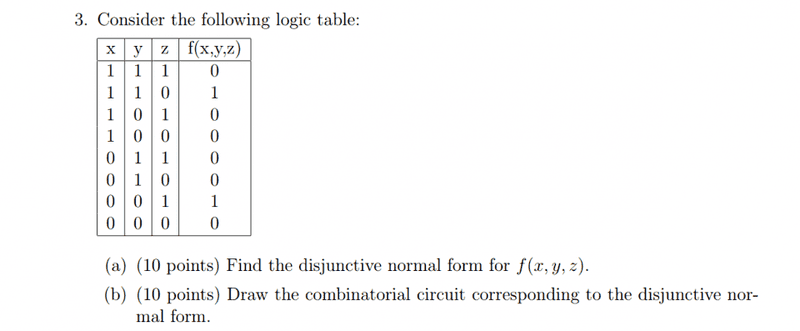# Disjunctive Normal form to Combinatorial Circuit

• Kingyou123

## Homework Statement

Draw the combinatorial circuit corresponding to the disjunctive normal form.## Homework Equations

DNF of f(x,y,z)=xyz+Not(xy)z

## The Attempt at a Solution

f(x,y,z)=xyz+not(xy)z
=z(xy+not(xy))
Wouldn't the xy and not xy cancel out? That's my current problem with this probelm.

The problem is that your DNF is not correct. Presumably the first term is supposed to give the 1 in the second row of the last column. But z is 0 in that row. So why are none of x,y,z negated in the term?
Your second term is not correct either. Are you aware that not(xy) is not the same as not(x)not(y)? de Morgan's Laws can sort that out for you.

The problem is that your DNF is not correct. Presumably the first term is supposed to give the 1 in the second row of the last column. But z is 0 in that row. So why are none of x,y,z negated in the term?
Your second term is not correct either. Are you aware that not(xy) is not the same as not(x)not(y)? de Morgan's Laws can sort that out for you.
Oh thank you for catching my mistake, I was looking at row 0 for some reason.
So I'm left with xynot(z) + not(x)not(y)z, wouldn't everything just cancel out?

wouldn't everything just cancel out?
No. In fact the expression cannot be factorized at all.